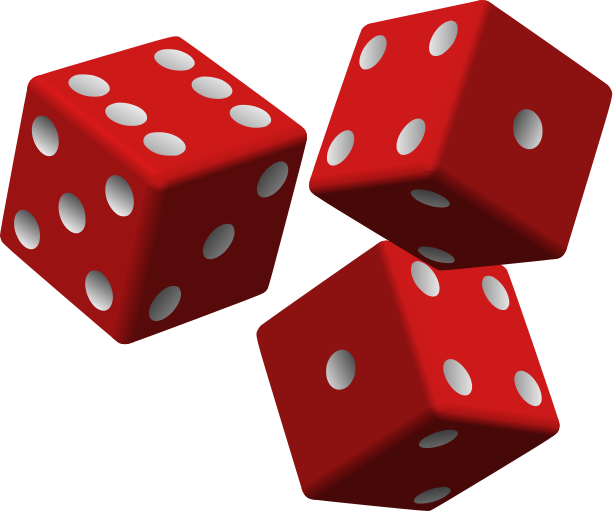# Dice O' Dice!

Probability Level 2Find the probability of getting at least two $( 2 )$ $4's$ in a roll of three $( 3)$ dice. Suppose the answer can be expressed as $\frac{a}{b}$ Find $a + b$.

You can try My Other Problems.

×